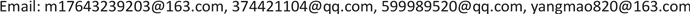SGSmart Grid2161-8763Scientific Research Publishing10.12677/SG.2019.92004SG-29500SG20190200000_95401591.pdf工程技术 基于奇异谱分析去噪和加权系数动态修正的风电功率实时组合预测模型 Real Time Combination Forecasting Model of Wind Power Based on Singular Spectrum Denoising Analysis and Dynamic Correction of Weighting Coefficients 21冠强31本明4121东北电力大学电气工程学院，吉林 国网临沂供电公司，山东 临沂国网吉林省电力科学研究院，吉林 长春null2803201909023140© Copyright 2014 by authors and Scientific Research Publishing Inc. 2014This work is licensed under the Creative Commons Attribution International License (CC BY). http://creativecommons.org/licenses/by/4.0/

1东北电力大学电气工程学院，吉林

2国网吉林省电力科学研究院，吉林 长春

3国网临沂供电公司，山东 临沂Copyright © 2019 by author(s) and Hans Publishers Inc.

This work is licensed under the Creative Commons Attribution International License (CC BY).

http://creativecommons.org/licenses/by/4.0/1. 引言

2. 奇异谱分析

1) 嵌入。设长度为N的一维时间序列 F N = ( f 1 , f 2 , ⋅ ⋅ ⋅ , f N ) ，给定正整数L为滑动窗口长度， 1 < L < N ，建立轨迹矩阵，可以表示为：

X = [ f 1 f 2 ⋅ ⋅ ⋅ f K f 2 f 3 ⋅ ⋅ ⋅ f K + 1 ⋮ ⋮ ⋮ ⋮ f L f L + 1 ⋅ ⋅ ⋅ f N ] (1)

2) 奇异谱分解。对轨迹矩阵X进行奇异谱分解，得到：

X = ∑ i = 1 d λ i U i V i T (2)

3) 分组。通常认为前r (r < d)个较大奇异值反映有用信号成分，后d - r个较小的奇异值被认为是噪声成分。这样，分组的操作就是确定合适的r值，从而实现信噪分离。

4) 对角平均化。实现矩阵转化为一维时间序列 的功能。重构序列可通过下式(3)获得，原序列由重构部分叠加如下式(4)计算得到：

y r c k = { 1 k ∑ m = 1 k y m , k − m + 1 ∗ 1 ≤ k < L ∗ 1 L ∗ ∑ m = 1 L * y m , k − m + 1 ∗ L ∗ ≤ k ≤ K ∗ 1 N − k + 1 ∑ m = k − K * + 1 N − K * + 1 y m , k − m + 1 ∗ K ∗ < k ≤ N (3)

y r = ∑ k = 1 L y r c k (4)

SSA在计算过程中有两个参数需要输入，即L和r，对重构序列进行分析确定 L = N / 2  ，此时重构误差最小；原始信号的功率谱密度的主频谱个数n，选取 r = 2 n  。SSA能够对时间序列进行分解，将最可预报的分量集中分布到若干个具有重要意义的分量中进行重建，将噪声信号筛选出，从而实现信噪分离。

3. 加权系数动态修正组合模型

P t ^ = p 1 t + p 2 t + ⋯ + p n t n (5)

P t ^ = c 1 P t 1 + ⋯ + c n P t n + c t (6)

Q = min ∑ j = 1 d ( T j t − P j t ^ ) 2 (7)

Q = min ∑ j = 1 d ( T j t − c t 1 P j t 1 − ⋯ − c t n P j t n − c t ) 2 (8)

ct为第t时刻的预测组合权值，求取Q对ct的极值点，下一时刻，更新历史数据，重新计算权值，实现权值的动态实时修正，保持组合效果最佳状态，将计算得到的权值代回公式(7)，得到最终的风电出力预测结果。

Relative error of forecasting result

P192.535.767.47
P292.226.107.78
P388.968.4211.04

4. 基于奇异谱分析去噪和加权系数动态修正的风电功率实时组合预测模型

1) 原始风电功率时间序列F经过奇异谱分析的嵌入、分解、分组和对角平均化最终得到重构后的风电功率时间序列Y；

2) 对重构后的时间序列Y分别建立单一预测模型，各单一预测模型输出结果是 P t 1 , ⋯ , P t n ；

3) 根据 Q = min ∑ j = 1 d ( T j t − P j t ^ ) 2 建立第t预测时刻下组合权值的求解方程，其中 P j t ^ = c 1 P j t 1 + ⋯ + c n P j t n + c t ，风电实际出力 T t = { T j t , j = 1 , ⋯ , d } ，并求解出权值系数 c t 1 , ⋯ , c t n , c t 。

4) 将步骤(3)求得的第t预测时刻下的权值系数引入公式(6)，便可计算获得未来第t预测时刻下的组合预测结果；

5) 下一时刻重新启动奇异谱分解与组合预测模型，更新训练样本数据，重复步骤(1)至步骤(4)，对权值进行动态修正，完成下一时刻的预测。

5. 算例分析5.1. 数据来源

Installed capacity of wind far

5.2. 评价指标

1) 日平均预测计划曲线准确率r1

r 1 i = [ 1 − 1 16 ∑ k = 1 16 ( P M i k − P p i k C a p ) 2 ] × 100 % (9)

r 1 = 1 96 ∑ i = 1 96 r 1 i (10)

2) 全天预测结果均方根误差r2

r 2 = 1 96 × 16 ∑ i = 1 96 ∑ k = 1 16 ( P M i k − P P i k C a p ) 2 × 100 % (11)

3) 全天预测结果平均绝对误差r3

r 3 = 1 96 × 16 ∑ i = 1 96 ∑ k = 1 16 | P M i k − P P i k C a p | × 100 % (12)

r1i为第i次实时预测的准确率； P M i k 为第i次实时预测中第k个时刻的实际功率； P P i k 为第i次实时预测中第k个时刻的预测功率；Cap为风电场装机容量。

5.3. 实验结果

Forecasting error result

Forecasting error results of C wind far

P190.589.427.95
P290.269.748.31
P387.8712.3011.24

Forecasting error results of A wind far

Forecasting error results of B wind far

6. 结论